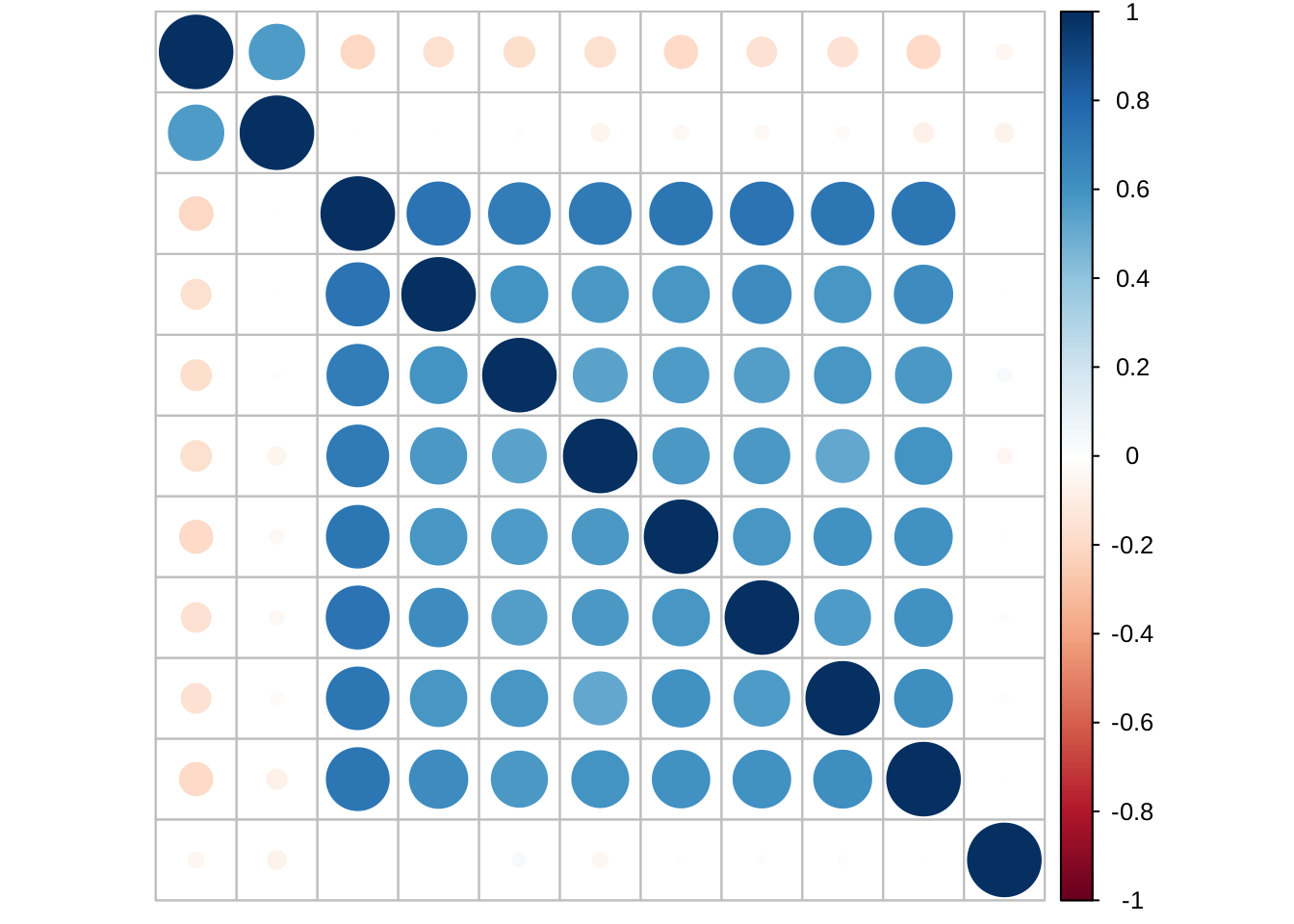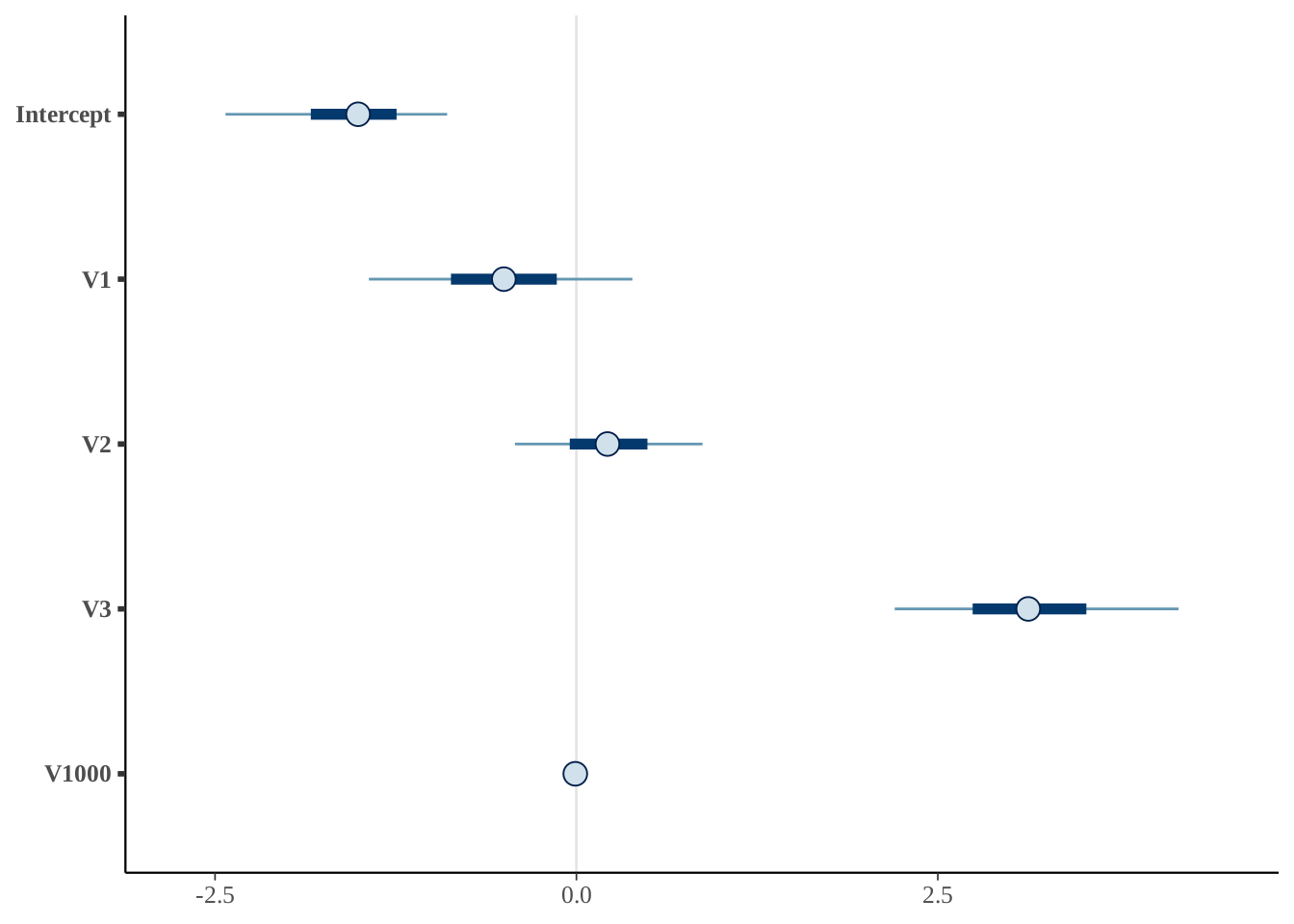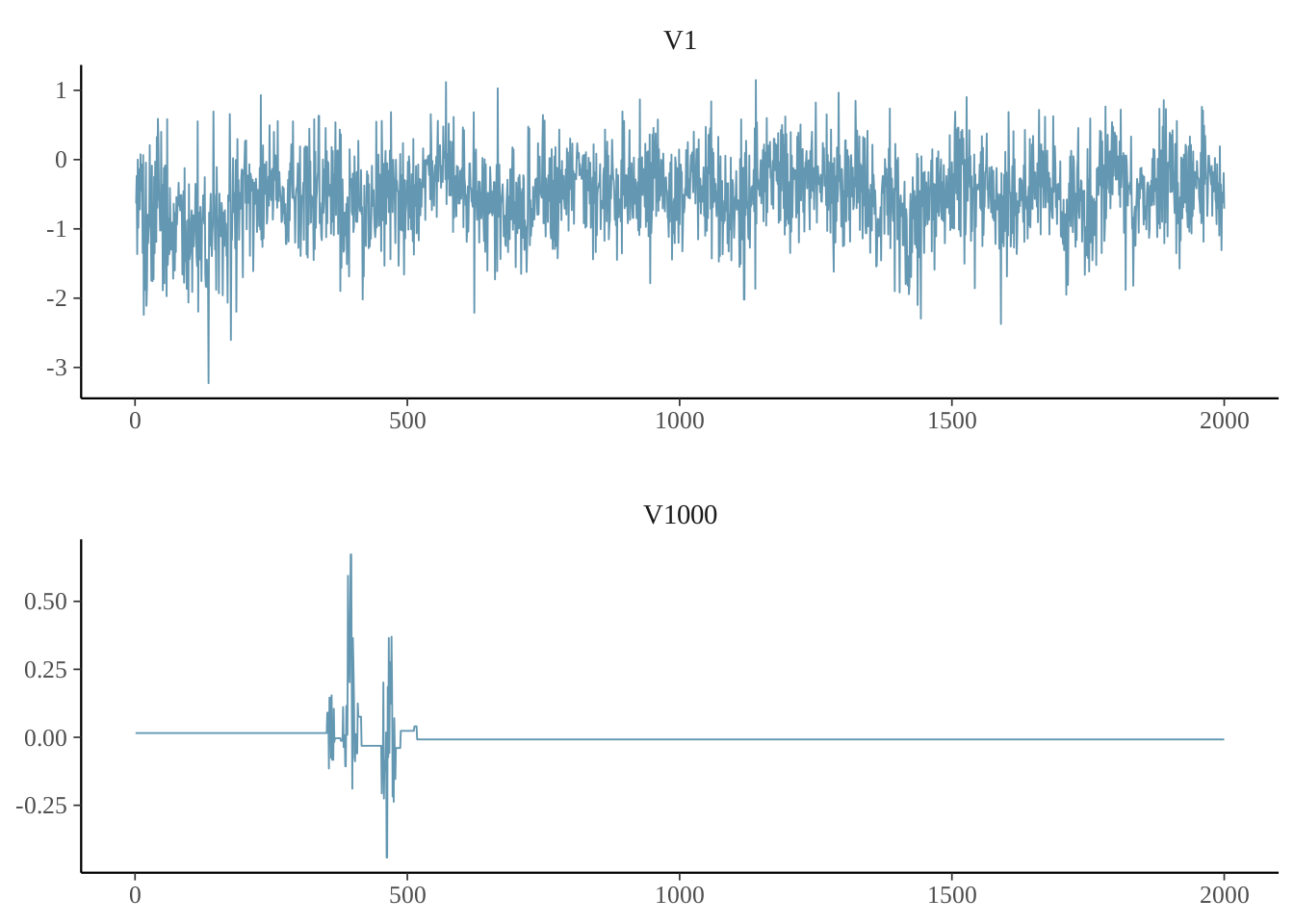# 1 Data Generation

``````library(HTLR)
library(bayesplot)
#> This is bayesplot version 1.7.0
#> - Online documentation and vignettes at mc-stan.org/bayesplot
#> - bayesplot theme set to bayesplot::theme_default()
#>    * Does _not_ affect other ggplot2 plots
#>    * See ?bayesplot_theme_set for details on theme setting``````

The description of the dataset generating scheme is found from Li and Yao (2018).

There are 4 groups of features:

• feature #1: marginally related feature

• feature #2: marginally unrelated feature, but feature #2 is correlated with feature #1

• feature #3 - #10: marginally related features and also internally correlated

• feature #11 - #2000: noise features without relationship with the y

``````SEED <- 101

n <- 510
p <- 2000

means <- rbind(
c(0, 1, 0),
c(0, 0, 0),
c(0, 0, 1),
c(0, 0, 1),
c(0, 0, 1),
c(0, 0, 1),
c(0, 0, 1),
c(0, 0, 1),
c(0, 0, 1),
c(0, 0, 1)
) * 2

means <- rbind(means, matrix(0, p - 10, 3))

A <- diag(1, p)

A[1:10, 1:3] <-
rbind(
c(1, 0, 0),
c(2, 1, 0),
c(0, 0, 1),
c(0, 0, 1),
c(0, 0, 1),
c(0, 0, 1),
c(0, 0, 1),
c(0, 0, 1),
c(0, 0, 1),
c(0, 0, 1)
)

set.seed(SEED)
dat <- gendata_FAM(n, means, A, sd_g = 0.5, stdx = TRUE)
str(dat)
#> List of 4
#>  \$ X  : num [1:510, 1:2000] -1.174 1.262 -0.752 -0.483 1.929 ...
#>   ..- attr(*, "dimnames")=List of 2
#>   .. ..\$ : NULL
#>   .. ..\$ : chr [1:2000] "V1" "V2" "V3" "V4" ...
#>  \$ y  : int [1:510] 1 2 3 1 2 3 1 2 3 1 ...
#>  \$ muj: num [1:2000, 1:3] -0.456 0 -0.456 -0.376 -0.376 ...
#>  \$ SGM: num [1:2000, 1:2000] 0.584 0.597 0 0 0 ...``````

Look at the correlation between features:

``````# require(corrplot)
cor(dat\$X[ , 1:11]) %>% corrplot::corrplot(tl.pos = "n")``````Split the data into training and testing sets:

``````set.seed(SEED)
dat <- split_data(dat\$X, dat\$y, n.train = 500)
str(dat)
#> List of 4
#>  \$ x.tr: num [1:500, 1:2000] -0.2476 0.0789 -1.5571 1.1904 -0.8982 ...
#>   ..- attr(*, "dimnames")=List of 2
#>   .. ..\$ : NULL
#>   .. ..\$ : chr [1:2000] "V1" "V2" "V3" "V4" ...
#>  \$ y.tr: int [1:500] 1 2 1 1 1 2 1 3 1 1 ...
#>  \$ x.te: num [1:10, 1:2000] 0.136 -0.634 -1.132 -0.283 0.188 ...
#>   ..- attr(*, "dimnames")=List of 2
#>   .. ..\$ : NULL
#>   .. ..\$ : chr [1:2000] "V1" "V2" "V3" "V4" ...
#>  \$ y.te: int [1:10] 2 2 3 3 3 1 1 3 2 3``````

# 2 Model Fitting

Fit a HTLR model with all default settings:

``````set.seed(SEED)
system.time(
fit.t <- htlr(dat\$x.tr, dat\$y.tr)
)
#>    user  system elapsed
#> 111.950   0.175  20.913
print(fit.t)
#> Fitted HTLR model
#>
#>  Data:
#>
#>   response:  3-class
#>   observations:  500
#>   predictors:    2001 (w/ intercept)
#>   standardised:  TRUE
#>
#>  Model:
#>
#>   prior dist:    t (df = 1, log(w) = -10.0)
#>   init state:    lasso
#>   sample:    1000 (posterior sample size)
#>
#>  Estimates:
#>
#>   model size:    5 (w/ intercept)
#>   coefficients: see help('summary.htlrfit')``````

With another configuration:

``````set.seed(SEED)
system.time(
fit.t2 <- htlr(X = dat\$x.tr, y = dat\$y.tr,
prior = htlr_prior("t", df = 1, logw = -20, sigmab0 = 1500),
iter = 4000, init = "bcbc")
)
#>    user  system elapsed
#> 159.698   0.447  28.243
print(fit.t2)
#> Fitted HTLR model
#>
#>  Data:
#>
#>   response:  3-class
#>   observations:  500
#>   predictors:    2001 (w/ intercept)
#>   standardised:  TRUE
#>
#>  Model:
#>
#>   prior dist:    t (df = 1, log(w) = -20.0)
#>   init state:    bcbc
#>   sample:    2000 (posterior sample size)
#>
#>  Estimates:
#>
#>   model size:    5 (w/ intercept)
#>   coefficients: see help('summary.htlrfit')``````

# 3 Model Inspection

Look at the point summaries of posterior of selected parameters:

``````summary(fit.t2, features = c(1:10, 100, 200, 1000, 2000), method = median)
#>                 class 2       class 3
#> Intercept -3.0617042129 -1.5103399543
#> V1         9.4541085933 -0.5020662323
#> V2        -5.7603486493  0.2149368251
#> V3         0.0359357704  3.1257249469
#> V4        -0.0034134708 -0.0004150318
#> V5        -0.0021260393 -0.0021705510
#> V6         0.0005933266  0.0014395451
#> V7        -0.1997239891  1.3111777939
#> V8        -0.0178842305 -0.0141715819
#> V9         0.0043794662 -0.0013018003
#> V10       -0.0004814938 -0.0063657810
#> V100      -0.0144196457 -0.0105803810
#> V200       0.0033995051 -0.0006334967
#> V1000     -0.0007550395 -0.0078158975
#> V2000     -0.0013167362  0.0002088031
#> attr(,"stats")
#>  "median"``````

Plot interval estimates from posterior draws using bayesplot:

``````post.t <- as.matrix(fit.t2, k = 2)
## signal parameters
mcmc_intervals(post.t, pars = c("Intercept", "V1", "V2", "V3", "V1000"))``````Trace plot of MCMC draws:

``mcmc_trace(post.t, c("V1", "V1000"), facet_args = list("nrow" = 2))``The coefficient of unrelated features (noise) are not updated during some iterations due to restricted Gibbs sampling Li and Yao (2018), hence the computational cost is greatly reduced.

# 4 Make Predictions

A glance at the prediction accuracy:

``````y.class <- predict(fit.t, dat\$x.te, type = "class")
y.class
#>       y.pred
#>  [1,]      2
#>  [2,]      2
#>  [3,]      3
#>  [4,]      1
#>  [5,]      3
#>  [6,]      1
#>  [7,]      1
#>  [8,]      3
#>  [9,]      2
#> [10,]      3
print(paste0("prediction accuracy of model 1 = ",
sum(y.class == dat\$y.te) / length(y.class)))
#>  "prediction accuracy of model 1 = 0.9"

y.class2 <- predict(fit.t2, dat\$x.te, type = "class")
print(paste0("prediction accuracy of model 2 = ",
sum(y.class2 == dat\$y.te) / length(y.class)))
#>  "prediction accuracy of model 2 = 1"``````

More details about the prediction result:

``````predict(fit.t, dat\$x.te, type = "response") %>%
evaluate_pred(y.true = dat\$y.te)``````